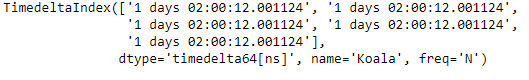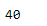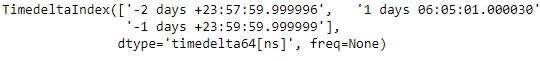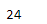# Python | Pandas TimedeltaIndex.nbytes

Python is a great language for doing data analysis, primarily because of the fantastic ecosystem of data-centric python packages. Pandas is one of those packages and makes importing and analyzing data much easier.

Pandas` TimedeltaIndex.nbytes` attribute return the number bytes occupied by the TimedeltaIndex object.

Syntax : TimedeltaIndex.nbytes

Return : size of the object in bytes

Example #1: Use `TimedeltaIndex.nbytes` attribute to find out the number of bytes occupied by the TimedeltaIndex object.

 `# importing pandas as pd ` `import` `pandas as pd ` ` `  `# Create the TimedeltaIndex object ` `tidx ``=` `pd.TimedeltaIndex(start ``=``'1 days 02:00:12.001124'``, periods ``=` `5``, ` `                                             ``freq ``=``'N'``, name ``=``'Koala'``) ` ` `  `# Print the TimedeltaIndex ` `print``(tidx) `

Output :Now we will print the number bytes taken by tidx object.

 `# find the size of tidx object in bytes ` `tidx.nbytes `

Output :As we can see in the output, the `TimedeltaIndex.nbytes` attribute has returned 40 indicating that tidx object is occupying this size memory block.

Example #2: Use `TimedeltaIndex.nbytes` attribute to find out the nanoseconds value for each entry of the TimedeltaIndex object.

 `# importing pandas as pd ` `import` `pandas as pd ` ` `  `# Create the TimedeltaIndex object ` `tidx ``=` `pd.TimedeltaIndex(data ``=``[``'-1 days 2 min 3us 10ns'``, ``'1 days 06:05:01.000030'``, ` `                                                      ``'-1 days + 23:59:59.999999'``]) ` ` `  `# Print the TimedeltaIndex ` `print``(tidx) `

Output :Now we will print the number bytes taken by tidx object.

 `# find the size of tidx object in bytes ` `tidx.nbytes `

Output :As we can see in the output, the `TimedeltaIndex.nbytes` attribute has returned 24 indicating that tidx object is occupying this size memory block.

My Personal Notes arrow_drop_upCheck out this Author's contributed articles.

If you like GeeksforGeeks and would like to contribute, you can also write an article using contribute.geeksforgeeks.org or mail your article to contribute@geeksforgeeks.org. See your article appearing on the GeeksforGeeks main page and help other Geeks.

Please Improve this article if you find anything incorrect by clicking on the "Improve Article" button below.

Article Tags :

Be the First to upvote.

Please write to us at contribute@geeksforgeeks.org to report any issue with the above content.